# Result and remainder

After dividing the unknown number by the number 23, the quotient 11 and the remainder 4 are formed. Find an unknown number.

Correct result:

x =  257

#### Solution: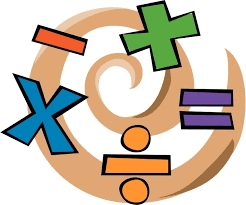We would be pleased if you find an error in the word problem, spelling mistakes, or inaccuracies and send it to us. Thank you!Tips to related online calculators
Do you want to perform natural numbers division - find the quotient and remainder?

## Next similar math problems:

• DivisibilityIs the number 761082 exactly divisible by 9? (the result is the integer and/or remainder is zero)
• Reminder and quotientThere are given numbers A = 135, B = 315. Find the smallest natural number R greater than 1 so that the proportions R:A, R:B are with the remainder 1.
• RemaindersIt is given a set of numbers { 170; 244; 299; 333; 351; 391; 423; 644 }. Divide this numbers by number 66 and determine set of remainders. As result write sum of this remainders.
• Unknown numberSamuel wrote unknown number. Then he had add 200000 to the number and the result multiply by three. When it calculated he was surprised, because the result would have received anyway, if write digit to the end of original number. Find unknown number.
• Sports studentsThere are 120 athletes, 48 volleyball players, and 72 handball players at the school with extended sports training. Is it possible to divide sports students into groups so that the number in each group is the same and expressed by the largest possible num
• Unknown numberIf I reduced the sum of the numbers 70 and the unknown number three times, I would get 100. what is the unknown number?
• Reminder and quotientThere are given the number C = 281, D = 201. Find the highest natural number S so that the C:S, D:S are with the remainder of 1,
• Quotient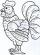Determine the quotient (q) and the remainder (r) from division numbers 100 and 8. Take the test of correctness.
• Divisible by nineHow many three-digit natural numbers in total are divisible without a remainder by the number 9?
• GroupsIn the 6th class there are 60 girls and 72 boys. We want to divide them into groups so that the number of girls and boys is the same. How many groups can you create? How many girls will be in the group?
• Big number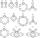hat is the remainder when dividing number 10 to 47 - 111 by number 9?
• Year 2020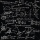The four-digit number divided by 2020 gives a result of 1, **. (Can not be in form 1,*0. ) Write all the options.
• RemainderWhat is the remainder of the division of natural numbers 293 and 7?
• NumbersDetermine the number of all positive integers less than 4183444 if each is divisible by 29, 7, 17. What is its sum?
• Sports games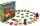Pupils of same school participated district sports games. When dividing into teams found that in the case of the creation teams with 4 pupils remaining 1 pupil, in the case of a five-member teams remaining 2 pupils and in the case of six-members teams rem
• Three Titanics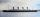Three steamers sailed from the same port on the same day. The first came back on the third day, fourth 4th day and the third returned sixth day. How many days after leaving the steamers met again in the harbor?
• Cash back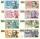After a trip 24 students have left more than 650 but less than 690 CZK (Kc). How much each student receives return when the amount is divisible by the number of pupils?